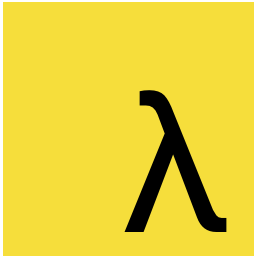# 30 天精通 RxJS (02)：Functional Programming 基本觀念

Dec 18th, 2016. 9 mins read

Functional Programming 是 Rx 最重要的觀念之一，基本上只要學會 FP 要上手 Rx 就不難了！Functional Programming 可以說是近年來的顯學，各種新的函式編程語言推出之外，其他舊有的語言也都在新版中加強對 FP 的支援！

## 什麼是 Functional Programming ?Functional Programming 是一種編程範式(programming paradigm)，就像 Object-oriented Programming(OOP)一樣，就是一種寫程式的方法論，這些方法論告訴我們如何思考及解決問題。

(5 + 6) - 1 * 3

const add = (a, b) => a + b
const mul = (a, b) => a * b
const sub = (a, b) => a - b

sub(add(5, 6), mul(1, 3))

## Functional Programming 基本要件

### 函式為一等公民 (First Class)

var hello = function() {}

fetch('www.google.com')
.then(function(response) {}) // 匿名 function 被傳入 then()

var a = function(a) {
return function(b) {
return a + b;
};
// 可以回傳一個 function
}

## Functional Programming 重要特性

### Expression, no Statement

Functional Programming 都是 表達式 (Expression) 不會是 陳述式(Statement)。 基本區分表達式與陳述式：

add(1,2)
• 陳述式 則是表現某個行為，例如一個 賦值給一個變數
a = 1;

### Pure Function

Pure function 是指 一個 function 給予相同的參數，永遠會回傳相同的返回值，並且沒有任何顯著的副作用(Side Effect)

var arr = [1, 2, 3, 4, 5];

arr.slice(0, 3); // [1, 2, 3]

arr.slice(0, 3); // [1, 2, 3]

arr.slice(0, 3); // [1, 2, 3]

var arr = [1, 2, 3, 4, 5];

arr.splice(0, 3); // [1, 2, 3]

arr.splice(0, 3); // [4, 5]

arr.slice(0, 3); // []

Side Effect

Side Effect 是指一個 function 做了跟本身運算返回值沒有關係的事，比如說修改某個全域變數，或是修改傳入參數的值，甚至是執行 console.log 都算是 Side Effect。

Functional Programming 強調沒有 Side Effect，也就是 function 要保持純粹，只做運算並返回一個值，沒有其他額外的行為。

• 發送 http request
• 在畫面印出值或是 log
• 獲得使用者 input
• Query DOM 物件

Referential transparency

### 利用參數保存狀態

function countReducer(state = 0, action) {
// ...
}

function findIndex(arr, predicate, start = 0) {
if (0 <= start && start < arr.length) {
if (predicate(arr[start])) {
return start;
}
return findIndex(arr, predicate, start+1);
}
}
findIndex(['a', 'b'], x => x === 'b'); // 找陣列中 'b' 的 index

## Functional Programming 優勢

### 可讀性高

[9, 4].concat([8, 7]) // 合併陣列
.sort()  // 排序
.filter(x => x > 5) // 過濾出大於 5 的

### 易於併行/平行處理

Functional Programming 易於做併行/平行(Concurrency/Parallel)處理，因為我們基本上只做運算不碰 I/O，再加上沒有 Side Effect 的特性，所以較不用擔心 deadlock 等問題。

Prev

Next